# NCERT solutions for Class 8 Maths Chapter 16 Playing with Numbers

NCERT Solutions for Class 8 Maths Chapter 16 Playing with Numbers- Maths is all about the numbers and you can simply play with the numbers. You have studied various types of numbers such as integer numbers, natural numbers, whole numbers, and rational numbers. You must have also studied interesting properties about them. In CBSE NCERT solutions for class 8 maths chapter 16 playing with numbers, you will find some interesting tricks to find divisibility of numbers. Important topics like divisibility by 10, 5, 2, 9 and 3, numbers in general form, letters for digits are covered in this chapter. In solutions of NCERT for Class 8 Maths Chapter 16 Playing with Numbers, there are some games with numbers and puzzles on the arithmetic problems given in the textbook which makes this chapter very interesting. To strengthen the foundation of the maths you must develop the ability to play with the numbers. For that, you should practice questions given in the textbook. If you are facing problem while solving exercises of NCERT textbook. You can take help from CBSE NCERT solutions for class 8 maths chapter 16 playing with numbers that are prepared and explained in a detailed manner. You can get NCERT solutions by clicking the below link.

Let’s take an example of games with numbers- two friends Meenakshi and Sundaram play a game Minakshi asks Sundaram to think a two-digit number and then-## Topics of NCERT Class 8 Maths Chapter 16 Playing with Numbers-

• 16.1 Introduction
• 16.2 Numbers in General Form
• 16.3 Games with Numbers
• 16.4 Letters for Digits
• 16.5 Tests of Divisibility

NCERT solutions for class 8 maths chapter 16 playing with numbers for all exercises

## Solutions of NCERT for class 8 maths chapter 16 playing with numbers topic 16.2 numbers in general form

Question:

25

any two digit number ab made of digits a and b can be written as ab = 10 × a + b = 10a + b

hence,

25 = 10*2 + 5 = 20 + 5

Question:

73

any two digit number ab made of digits a and b can be written as ab = 10 × a + b = 10a + b

hence generalized form of the number

73 = 10*7 + 3

Question:1(iii)

129

A 3 digit number madeup of digits a, b, c will be written as,

abc = 100 × a + 10 × b + 1 × c = 100a + 10b + c

hence generalised form of number

129 = 100*1 + 10*2 + 1*9

Question:

302

A 3-digit number abc made up of digits a, b and c is written as abc = 100 × a + 10 × b + 1 × c = 100a + 10b + c

hence generalised form of

302 = 100*3 + 10*0 + 1*2

Question:2(i)

As we know ab = 10 × a + b = 10a + b

usual form of number

10*5 + 6 = 50 + 6 = 56

Question:

As we know abc = 100 × a + 10 × b + 1 × c

the usual form of number

100*7 + 10*1 + 8 = 700 + 10 + 8 = 718.

Question:2(iii)

As we know abc = 100 × a + 10 × b + 1 × c

casual form of 100 × a + 10 × b + 1 × c = abc.

CBSE NCERT solutions for class 8 maths chapter 16 playing with numbers topic 16.3 games with numbers

27

As we know

(10a + b) + (10b + a) = 11a + 11b = 11 (a + b)

here a = 2 and b = 7

27 + 72 = 99= 11 *9 =  a multiple of 11

39

39 + 93 = 133 = 11*12 = a multiple of 11

this can be explained by

(10a + b) + (10b + a) = 11a + 11b = 11 (a + b)

Here a = 3 and b = 9

64

64 + 46 = 110 = 11*10= a multiple of 11

this can be explained by

(10a + b) + (10b + a) = 11a + 11b = 11 (a + b)

here a = 6 and b = 4

17

17 + 71 = 88 = 11*8 = a multiple of 11

this can be explained by

(10a + b) + (10b + a) = 11a + 11b = 11 (a + b)

here a = 1 and b = 7

Solutions of NCERT for class 8 maths chapter 16 playing with numbers topic 16.3 games with numbers

17

If the tens digit is larger than the ones digit (that is, a > b), then
(10a + b) – (10b + a) = 10a + b – 10b – a
= 9a – 9b = 9(a – b).
If the unit digit is larger than the tens digit (that is, b > a), he does:
(10b + a) – (10a + b) = 9(b – a).

here a = 1 and b = 7

71 - 17 = 54 = 9*6 = multiple of 9

21

If the tens digit is larger than the ones digit (that is, a > b), then
(10a + b) – (10b + a) = 10a + b – 10b – a
= 9a – 9b = 9(a – b).
If the unit digit is larger than the tens digit (that is, b > a), he does:
(10b + a) – (10a + b) = 9(b – a).

here a = 2 and b = 1

21 - 12 = 9 = 9*1 = multiple of 9

96

If the tens digit is larger than the ones digit (that is, a > b), then
(10a + b) – (10b + a) = 10a + b – 10b – a
= 9a – 9b = 9(a – b).
If the unit digit is larger than the tens digit (that is, b > a), he does:
(10b + a) – (10a + b) = 9(b – a).

here a = 9 and b = 6

96 - 69 = 27= 9*3= multiple of 9

37

If the tens digit is larger than the ones digit (that is, a > b), then
(10a + b) – (10b + a) = 10a + b – 10b – a
= 9a – 9b = 9(a – b).
If the unit digit is larger than the tens digit (that is, b > a), he does:
(10b + a) – (10a + b) = 9(b – a).

here a = 3 and b = 7

73 - 37 = 36 = 9*4 = multiple of 9

Solutions of NCERT for class 8 maths chapter 16 playing with numbers topic 16.3 games with numbers

132

Let's assume the 3-digit number chosen by Minakshi = 100a + 10b + c.

After reversing the order of the digits, number = 100c + 10b + a.

On subtraction:

• If a > c, then the difference between the original number & reversed number

(100a + 10b + c) – (100c + 10b + a) = 100a + 10b + c – 100c – 10b – a = 99a – 99c = 99(a – c).

• If c > a, then the difference between the numbers is (100c + 10b + a) – (100a + 10b + c) = 99c – 99a = 99(c – a).

• If a & c are equal,then the difference is 0.

here a = 1, b = 3 and c = 2

231 - 132 = 99 = multiple of 99

469

Let the 3-digit number chosen by Minakshi = 100a + 10b + c.

After reversing the order of the digits, number = 100c + 10b + a.

On subtraction:

• If a > c, then the difference between the original numbedr & reversed numbers is

(100a + 10b + c) – (100c + 10b + a) = 100a + 10b + c – 100c – 10b – a = 99a – 99c = 99(a – c).

• If c > a, then the difference between the numbers is (100c + 10b + a) – (100a + 10b + c) = 99c – 99a = 99(c – a).

• if a & c are equal, then the difference is 0.

here a,b and c are 4, 6 & 9 respectively.

964 - 469 = 495 = 99*5 =  multiple of 99

737

Let the 3-digit number chosen by Minakshi = 100a + 10b + c.

After reversing the order of the digits, number = 100c + 10b + a.

On subtraction:

• If a > c, then the difference between the original number & reversed number is

(100a + 10b + c) – (100c + 10b + a) = 100a + 10b + c – 100c – 10b – a = 99a – 99c = 99(a – c).

• If c > a, then the difference between the numbers is (100c + 10b + a) – (100a + 10b + c) = 99c – 99a = 99(c – a).

• If A 7 C are equal, the difference is 0.

here a = 7, b = 3 and c = 7

737- 737 = 0= multiple of 99

901

Let the 3-digit number chosen by Minakshi = 100a + 10b + c.

After reversing the order of the digits, number = 100c + 10b + a.

On subtraction:

• If a > c, then the difference between the original number & reversed number is

(100a + 10b + c) – (100c + 10b + a) = 100a + 10b + c – 100c – 10b – a = 99a – 99c = 99(a – c).

• If c > a, then the difference between the numbers is (100c + 10b + a) – (100a + 10b + c) = 99c – 99a = 99(c – a).

• If a & c are equal, the difference is 0.

here a = 9, b = 0 and c = 1

901- 109= 792= 99*8 = multiple of 99

quotient in each case = c - a.

CBSE NCERT solutions for class 8 maths chapter 16 playing with numbers topic 16.3 games with numbers

417

Let choosen number be abc then,

abc = 100a + 10b + c

cab = 100c + 10a + b

bca = 100b + 10c + a

After adding all the above three, abc + cab + bca = 111(a + b + c) = 37 × 3(a + b + c),

It will be divisible by 37 becuase 37 is present in the equation.

here a = 4, b = 1, and c = 7

417 + 741 + 147 = 1332 = 37*36  i.e. divisible by 37.

632

Let choosen number be abc then,

abc = 100a + 10b + c

cab = 100c + 10a + b

bca = 100b + 10c + a

The addition of all the above three, abc + cab + bca = 111(a + b + c) = 37 × 3(a + b + c), which is divisible by 37

here a = 6, b = 3, and c = 2

632+ 263+ 362= 1221= 37*33 i.e. divisible by 37.

117

Let choosen number be abc then,

abc = 100a + 10b + c

cab = 100c + 10a + b

bca = 100b + 10c + a

The addition of the above all three, abc + cab + bca = 111(a + b + c) = 37 × 3(a + b + c), which is divisible by 37

here a = 1, b = 1, and c = 7

117 + 711 + 117 = 999 = 37*27  i.e. divisible by 37.

937

Let choosen number be abc then,

abc = 100a + 10b + c

cab = 100c + 10a + b

bca = 100b + 10c + a

The addition of all above three, abc + cab + bca = 111(a + b + c) = 37 × 3(a + b + c), which is divisible by 37

here a = 9, b = 3, and c = 7

937 + 793 + 397 = 2109 = 37*57  i.e. divisible by 37.

## Solutions of NCERT for class 8 maths chapter 16 playing with numbers-Exercise: 16.1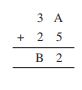Here we are adding two numbers and unit place of the first number and the second number is A and  5 respectively. unit place of the answer is 2 so the way we can get this result is when we get 12 on adding unit places of both number i.e.

A + 5 = 12

which implies      A = 12 - 5 = 7.

Ten's digit of both numbers are 3 and 2.remainder=1

so ten's digit of the answer(B) = 3 + 2 + 1 = 6

Hence A= 7 and B = 6.Here

answer's unit place = 3 , possible addition of unit places digit = 13

A + 8 = 13

A = 13 - 8 = 5

remainder = 1

Ten's place of answer  = 4 + 9 + 1 =  14

B = 4

remainder = 1

100's place = C = 1

Hence value of  A = 5 , B = 4 and C = 1.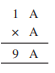Here

first clue :

we have A = a number which when multiplied by itself gives the same number in the unit digit.

possible numbers = 1 and 6

Second Clue:

number when multiplied with 1 and added with the reminder of previous multiplication( A*A) = 9

both first and second clue implies that A = 6.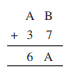There can be two cases

1. when the addition of unit place digit doesn't  produce Carry

A + 3 = 6

A = 3

However, to get 3 in unit place of our answer our B has to be 6 and that would produce carry hence this case is not possible.

2. when the addition of unit place digit produces Carry

A + 3 +1 = 6

A = 2

for getting 2 in unit place of answer we need the sum of unit digit of numbers = 12

B + 7 = 12

B = 5

Hence A = 2 and B = 5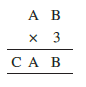Here multiplication of 3 and B gives  a number whose unit place digit is B .

Possible value of B = 0 and 5

let B = 5

3 * A  + 1 = CA

this is not possible for any value of A.

Hence B = 0

now  A * 3 = CA ( a number whose unit place digit is A itself when multiplied by 3) hence

possible value of A = 5 and 0

since AB is a two digit number A can not equal to 0.

hence A = 5

A * 3 + 1 = CA

5 * 3 + 1 = 15

hence C = 1

A = 5, B = 5 and C = 1.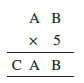Here , multiplication of B and 5 gives a number whose ones digit is B. this is possible when B = 0 or 5

let B = 5

B * 5 = 5 * 5 = 25

Carry = 2

5*A + 2 = CA  , This os possible only when A = 2 or 7

when A = 2 ,

5*2 + 2 = 12 which implies C = 1

when A = 7

5*7 + 2 = 37 which implies C = 3

now  B = 0

B*5 = 0*5 = 0

Carry = 0 so

5 * A = CA which is possible when A = 0 or 5 Howerver A cannot equal to 0 since AB is a two digit number

so A = 5

5*5 = 25 which implies C = 2

hence possible values of A B and C are

A = 5, 2, 7 , B = 0, 5, 5 and C = 2, 1, 3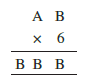The product of 6 & B gives a number whose unit digit is B again.

possible value of B = 0. 2 , 4, 6 or 8

If B = o then our product will be zero. hence this value of B is not possible.

If B = 2, then B x 6 = 12 . Carry for next step = 1.

6A + 1 = BB = 22

implies A = 21/6 = not any integer value hence this case is also not possible.

If B = 6  then B *6 = 36 and 3 will be carry for next step.

6A + 3 = BB = 66

implies A = 63/6 = not an integer value hence this case is also not possible.

If B = 8 then B * 6 = 48 and 4 will be carry for next step.

6A + 4 = BB = 88

implies A = 14 however A is a single digit number hence this case is also not possible.

If B = 4 then B*6 = 24 and 2 will be carry for next step.

6A + 2 = BB = 22

implies A = 7 .

Hence A = 7 and B = 4 is the correct answer.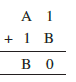The addition of 1 and B gives a number whose ones digit is 0. this is possible when digit B = 9 .

1 + B  = 10  and 1 is the Carry for the next step

Now, A + 1 + 1 = B => 9

Implies  A = 7.

Hence A = 7 and B = 9 is Correct answer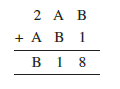The additiion of B and 1 is 8  is giving a number whose ones digit is 8. this means digit B is 7 .

B + 1 = 8 and no carry for next step.

next step :

Now, A + B = 1 => A + 7

which implies A = 4

A  + B = 11 and 1 is carry for next step

1 + 2 + A  = B

1 + 2 + 4 = 7

Hence A = 4 and B = 7 is correct answer.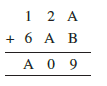The addition of A and B is giving a number whose ones digit is 9. The sum can only be 9 not 10 as a sum of two single digits cannot exceed 18. hence there will not be any carry for the next step

2 + A = 0

implies A = 8

2 + 8 = 10 and 1 is the carry for next step.

1 + 1 + 6 = A = 8

it satisfies hence A = 8 and B = 1 is the correct answer.

## Solutions of NCERT for class 8 maths chapter 16 playing with numbers topic 16.5.2 divisibility by 5

The detailed solution for the above-written problem is as follows,

The unit digit, when divided by 5, must be leaving a remainder of 3. So the unit digit must be either 3 or 8.

The detailed solution for the above-written question is as follows

If a number is divisible by 5 then it's unit digit must be 0 or 5. so if we need the remainder of 1 when divided by 5 then the numbers unit digit must be 1 or 6.

The detailed solution for the above-written question is as follows

If the unit digit of a number is 0 or 5, then it is divisible by 5. hence if we need the remainder of 4 then unit digit of number should be 4 or 9.

CBSE NCERT solutions for class 8 maths chapter 16 playing with numbers topic 16.5.3 divisibility by 2

The detailed solution for the above-written question is as follows

N is odd; so it's unit digit is odd. Therefore, the unit digit must be 1, 3, 5, 7 or 9.

The detailed solution for the above-written question is as follows

N is Even; so it's unit digit is even. Therefore, the unit digit must be 2, 4, 6, 8 or 0.

Since N leaves the remainder of 4 when divided by 5. the possible values in ones place of number N are 4 or 9.

now, since it leaves a remainder of 1 when divided by 2, the N would be an odd number. hence ones digit of N is also an odd number. which means ones digit of our number N is 9.

Solutions of NCERT for class 8 maths chapter 16 playing with numbers topic 16.5.4 divisibility by 9 and 3

108

Any number will be divisible by 9 only if the sum of all the digits in that number will be divisible by 9.

Sum of digit of 108 = 1 + 0 + 8 = 9 which is divisible by 9 i.e (9/9 = 1).

hence we conclude 108 is divisible by 9.

616

Any number will be divisible by 9 only if the sum of all the digits in that number will be divisible by 9.

Sum of digits of 616 = 6 + 1 + 6 = 13 which is not divisible by 9,

Hence we conclude 616 is not divisible by by 9.

294

Any number will be divisible by 9 only if the sum of all the digits in that number will be divisible by 9.

Sum of digits of 294 = 2 + 9 + 4 = 15 which is not divisible by 9.

Hence we conclude 294 is not divisible by 9.

432

Any number will be divisible by 9 only if the sum of all the digits in that number will be divisible by 9.

Sum of digit 432 = 4 + 3 + 2 = 9 which is divisible by 9.

Hence we conclude 432 is divisible by 9.

927

Any number will be divisible by 9 only if the sum of all the digits in that number will be divisible by 9.

Sum of digits of 927 = 9 + 2 + 7 = 18 which is divisible by 18.

Hence we conclude number 927 is divisible by 9.

Solutions of NCERT for class 8 maths chapter 16 playing with numbers topic think, discuss and write

Yes, it has been prooved that if a number is divisible by any number m, then it will also be divisible by each of the factors of m.

Let's Assume n is divisible by m, and m is divisible by k.This means

n=pm and

m = qk where all are integers

Now,

n = p(qk) =( pq)k which means n is divisible by k.

Hence a number is divisible by any number m, then it will also be divisible by each of the factors of m.

Is it necessary that   should be divisible by 11?

let the number abc be 132

Here a = 1, b = 3 and c = 2

132= 100*1 + 10*3 + 2 = 99 + 11*3 + (1 - 3 + 2)

= 11(9*1+3) + (1 - 3 + 2 )

if number is divisible by 11 then (a - b + c )  must be divisible by 11.

as in above case of number 132 the a - b + c = 1 -3 + 2 = 0 whichis divisible by 11.

Hence we conclude ( a - b + c ) should be divisible by 11 if abc is divisible by 11.

If the number abcd is divisible by 11 then [ (b + d) - (a + c) ] also must be divisible by 11.

let the number be 1089

here a = 1, b = 0, c = 8 and d = 9

1089 = 1000*1 + 100*0 + 10*8 + 9

= (1001*1 + 99*0 + 11*8) + [(0 + 9) - (1 + 8)]

= 11(91*1 + 9*0 + 8) + [ 9 - 9 ]

here  [ (b + d) - (a + c) ] = [9 - 9 ] = 0 which is divisible by 11.

hence If the number abcd is divisible by 11 then [ (b + d) - (a + c) ] also must be divisible by 11.

Yes,

A number will always be divisible by 11 if the difference between the sum of digits at its odd places and that of digits at the even places is divisible by 11.

So, for instance, 2728 has the alternating sum of digits 2-7+2-8 = -11. Here -11 is divisible by 11, so is 2728.

Similarly, for 31415, the alternating sum of digits is 3-1+4-1+5 = 10. This would not divisible by 11, so neither is 31415.

NCERT solutions for class 8 maths chapter 16 playing with numbers topic 16.5.4 divisibility by 9 and 3

108

Any number will be divisible by 3 only if the sum of all the digits in that number will be divisible by 3.

Sum of digits of number 108 = 1 + 0 +8 = 9 which is divisible by 3.

Hence we conclude number 108 is divisible by 3.

616

Any number will be divisible by 3 only if the sum of all the digits in that number will be divisible by 3.

Sum of digits of number 616 = 6 + 1 + 6 = 13 which is not divisible by 3.

Hence we conclude number 616 is not divisible by 3.

294

Any number will be divisible by 3 only if the sum of all the digits in that number will be divisible by 3.

Sum of digits of number 294= 2 + 9 + 4 = 15 which is divisible by 3.

Hence we conclude number 294 is divisible by 3.

432

Any number will be divisible by 3 only if the sum of all the digits in that number will be divisible by 3.

Sum of digits of number 432 = 4 + 3 + 2 = 9 which is divisible by 3.

Hence we conclude number 432 is divisible by 3.

927

Any number will be divisible by 3 only if the sum of all the digits in that number will be divisible by 3.

Sum of digits of number 927 = 9 + 2 + 7 = 18 which is divisible by 3.

Hence we conclude number 108 is divisible by 3.

## CBSE NCERT solutions for class 8 maths chapter 16 playing with numbers-Excercise: 16.2

If a number is a multiple of 9 , then the sum of its digit will be divisible by 9.

Sum of digits of 21y5= 2 + 1 + y + 5 = 8 + y

8 + y is a multiple of 9 when y = 1 (since y can only be single digit )

hence y = 1 is correct answer.

If a number is a multiple of 9, then the sum of its digit will be divisible by 9 .

Sum of digits of 31z5 = 3 + 1 + z + 5 = 9 + z

hence 9 + z have to be multiple of 9

this possible when 9 + z = 0, 9 , 18, 27 ...

since z is a single digit number this sum can only be 9 or 18 . therefore, z should be 0 or 9 .

hence two possible values of z = 0 or 9.

(Since is a multiple of 3, its sum of digits   is a multiple of 3; so is one of these numbers: 0, 3, 6, 9, 12, 15, 18, ... . But since is a digit, it can only be that . Therefore, . Thus, can have any of four different values.)

if a number is multiple of 3 then the sum of its digits is also a multiple of 3.

Summ of digits of 24x = 2 + 4 + x = 6 + x

6 + x is a multiple of 3 which means

6 + x = 0 or 3 or 9 or 12....

but since x is a single digit the possible sum is 6 or 9 or 12 or 15 and hence value of x are 0 or 3 or 6 or 9 respectively.

hence possible value of x = 0, 3, 6, or 9.

If a number is multiple of 3, the sum of its digits will be multiple of 3.

Sum of digits of 31z5 = 3 + 1 + z + 5 = 9 + z

9 + z is a multiple of 3 and since z is a single digit

9 + z  is any one of 9, 12, 15 or 18

thus value of z is 0, 3, 6 or 9 respectively.

Hence the possible value of z is 0,3,6 or 9.

NCERT solutions for class 8 maths: Chapter-wise

 Chapter -1 NCERT solutions for class 8 maths chapter 1 Rational Numbers Chapter -2 Solutions of NCERT for class 8 maths chapter 2 Linear Equations in One Variable Chapter-3 CBSE NCERT solutions for class 8 maths chapter 3 Understanding Quadrilaterals Chapter-4 NCERT solutions for class 8 maths chapter 4 Practical Geometry Chapter-5 Solutions of NCERT for class 8 maths chapter 5 Data Handling Chapter-6 CBSE NCERT solutions for class 8 maths chapter 6 Squares and Square Roots Chapter-7 NCERT solutions for class 8 maths chapter 7 Cubes and Cube Roots Chapter-8 Solutions of NCERT for class 8 maths chapter 8 Comparing Quantities Chapter-9 NCERT solutions for class 8 maths chapter 9 Algebraic Expressions and Identities Chapter-10 CBSE NCERT solutions for class 8 maths chapter 10 Visualizing Solid Shapes Chapter-11 NCERT solutions for class 8 maths chapter 11 Mensuration Chapter-12 Solutions of NCERT for class 8 maths chapter 12 Exponents and Powers Chapter-13 CBSE NCERT solutions for class 8 maths chapter 13 Direct and Inverse Proportions Chapter-14 NCERT solutions for class 8 maths chapter 14 Factorization Chapter-15 Solutions of NCERT for class 8 maths chapter 15 Introduction to Graphs Chapter-16 CBSE NCERT solutions for class 8 maths chapter 16 Playing with Numbers

## NCERT solutions for class 8: Subject-wise

There are some important short tricks from NCERT solutions for class 8 maths chapter 16 playing with numbers, to check divisibility of the numbers is listed below.

• A number is divisible by three if the sum of the digits if the number is divisible by three otherwise it is not divisible by three.

Example-  i) 313 -> The sum of digits=3+1+3=7 which is not divisible by 3. So 313 is not divisible by 3

ii) 3162 -> The sum of digits=3+1+6+2=12 which is divisible by 3. So 3162 is divisible by 3

• A number is divisible by nine if the sum of the digits if the number is divisible by nine otherwise it is not divisible by nine.

Example- i) 152875 -> The sum of digits=1+5+2+8+7+5=28 which is not divisible by 9. So 152875 is not divisible by 9

• A number is divisible by 5 If the one's digit of a number is 0 or 5 otherwise it is not divisible by 5
• A number is divisible by 2 If the one’s digit of a number is 0, 2, 4, 6 or 8 or otherwise it is not divisible by 2.

There are 2 exercises with 14 questions given in the textbook. Practice these questions to get conceptual clarity. You can take help from CBSE NCERT solutions for class 8 maths chapter 16 playing with numbers.

Happy learning!!!